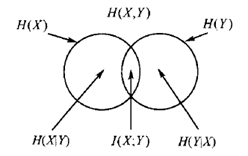## 信息量

$I= log_2(\frac{1}{p(x)})=-log_2(p(x))$ 就是对概率小的编码量就大，概率大的、使用频次高的信息编码大，这样，使得整个信息存储空间最小。所以，I通俗就是编码量，就是不确定性，混乱程度，都是一个意思。

## 熵

$H(X)=-\sum_xp(x)log_2(p(x))$ 信息量取期望，说白了就是衡量一下这个系统的平均混乱度。熵越大，混乱度越大，越不确定。那个曲线人人都知道，就不画了，熵在$p(x)$取x=0.5时候取得最大为1。

## 联合熵

$H(X,Y)=-\sum_xp(x,y)log_2(p(x,y))$ 这个没啥好说的，熵的两个随机变量的升级版，呵呵

## 条件熵

$H(X|Y)=-\sum_{x\in X,y\in Y}p(x,y)log(p(x|y)$ 为何前面是p(x,y)，而不是条件概率呢？

## 互信息

$I(X;Y)=\sum_{x\in X,y \in Y}log\frac{p(x,y)}{p(x)p(y)}$ $I(X;Y)=H(X)-H(X|Y)$ 互信息就是，Y这个变量引入后，X变量的熵的减少量。这很容易得出一个结论，如果X,Y是相互独立，那么互信息就是0。

熵、联合熵、条件熵、互信息的关系……………………我是分割线…………………..

交叉熵和KL散度和上面的信息熵、互信息和条件熵完全不是一码事，它们都是在谈论1个或者几个随机变量，但是交叉熵和KL散度，谈的则是一个随机变量，但是有多个概率分布，这些概率分布之间的差异大小就是靠KL散度（相对熵）和交叉熵来描述的


## 交叉熵

$H(p,q)=-\sum_xp(x)logq(x)$ 看！看！只有一个随机变量x，而p,q则是关于x的两种分布 $=\sum_xp(x)log\frac{1}{q(x)}$看！看！这个是拿q来编码p，如果不理解，看最最上面关于信息量的解释

## KL散度（相对熵）

$D_{KL}(A||B)=\sum_ip(x_i)log( \frac{p(x_i)}{q(x_i)})$

信息熵、交叉熵、KL散度的关系

$D_{KL}(A||B)=\sum_ip(x_i)log( \frac{p(x_i)}{q(x_i)})$ $=\sum_ip(x_i)logp(x_i)-\sum_ip(x_i)logq(x_i)$ $=H(p)-H(p,q)$ 看！就是p的信息熵减去p、q的交叉熵呀这里有个问题：为什么机器学习中，都用交叉熵，而不用KL散度？

$D_{KL}(A||B) = -H(A)+H(A,B)$ 如果 $H(A)$是一个常量，那么 $D_{KL}(A||B) = H(A,B)$ ，也就是说KL散度和交叉熵在特定条件下等价。这个发现是这篇回答的重点。 既然等价，那么我们优先选择更简单的公式，因此选择交叉熵。